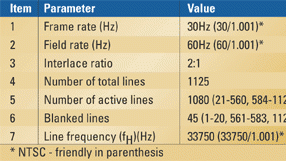# The 1920x1080/60i HDTV formatThe picture scanning characteristics

Table 1. Picture scanning characteristics of the 1920x1080/60i format are listed above.

Table 1 shows that the 1920x1080/60i format has a nominal frame rate of 30Hz or 30/1.001Hz (NTSC-friendly). Each frame consists of two interlaced fields with an interlace ratio of 2:1, resulting in a field rate of 60Hz, or 60/1.001Hz for NTSC-friendly applications.

There are a total of 1125 lines per frame. In an analog system this would imply 562.5 lines in each field. However, because a digital interface has to be supported, only whole numbers of lines in each field are allowed. This permits the unambiguous identification of the lines by the digital timing reference sequences (TRS). As a consequence, the interlace versions define integer, and hence unequal, numbers of lines in each of the two fields comprising a frame. Field 1 has 563 lines and Field 2 has 562 lines. The horizontal scanning frequency is 33750Hz. It is interesting to note that this format uses analog sync signals that differ from those used by SDTV formats. The analog sync signals are not digitized, so they are rarely, if ever, encountered in practice. The reader is referred to the relevant SMPTE or ITU standard for details.

The digital representation

The digital coding is based on one luminance (E´Y) and two color-difference (E´CB and E´CR) analog signals, or on the use of the three primary analog signals – E´G, E´B and E´R. This article will deal with some aspects of the 1920x1080/60i format using E´Y, E´CB and E´CR analog source signals.

The coded signals’ matrix coefficients are defined in ITU-R BT.709 and are significantly different from those specified by ITU-R BT.601. The implication here is that format conversion applications require matrixing recalculation.

The luminance sampling frequency of 74.25MHz is obtained from the analog input video sync signal using a PLLC oscillator operating at 2200 x fH. This results in a Nyquist frequency of 37.125MHz. The specified anti-aliasing low-pass filter has a cutoff frequency of 30MHz. The color-difference signals’ sampling frequency is 37.25MHz or 1100 x fH. This results in a Nyquist frequency of 18.5625MHz. The specified anti-aliasing low-pass filter has a cutoff frequency of 15MHz. The selected sampling frequencies result in an active line with 1920 Y samples and 960 each CB and CR samples.

As shown in Figure 1, the digital representation assumes two separate bit-parallel datastreams consisting of:

• A digital datastream conveying a digitized luminance bit-parallel signal Y with a data rate of 74.25Mwords/s.
• A digital datastream conveying digitized time-division-multiplexed bit-parallel signals CB and CR with a data rate of 74.25Mwords/s.

Table 2. A digital representation of the 1920x1080/60i format is provided.

Each datastream carries the active video information, as well as its own TRS information (EAV and SAV) and the ancillary data if present. In a 10-bit system the digital information occupies a range extending from 000h to 3FFh (0 to 1023 decimal). Table 2 shows that the luminance (Y) signal normally extends from black [040h (64)] to white [3ACh (940)]. The CB and CR signals normally extend from 040h (64) to 3C0h (960). In order to cater to overshoot and undershoot, the allowed range is extended to 004h to 3FBh (4 to 1019). Values from 000h to 003h (0 to 3) and 3FCh to 3FFh (1020 to 1023) are reserved for TRS signals (EAV and SAV).

The end of active video (EAV) and start of active video (SAV) signals each consist of a four-word sequence:

• The three synchronizing words with hexadecimal values of, respectively, 3FF, 000 and 000.
• The XYZ word that carries the V, F and H bits, which define the vertical and horizontal blanking. In addition, bits P0, P1, P2 and P3, which assume values depending on the status of the V, F and H bits, provide limited error correction (single errors) and detection (two errors) of these bits.

Figure 2. The typical frequency response of the CB, CR and Y channels is illustrated in this figure.

Resolution considerations

The vertical resolution, expressed in “lines per picture height” (LPH), is equal to the number of active lines (1080) multiplied by the controversial Kell factor, taken as 0.7. So the 1920x1080/60i format has a vertical resolution of 1080 x 0.7 = 756 LPH. Given the active line duration, the horizontal resolution factor is 29 lines/MHz. With the specified anti-alias filter with a cutoff frequency of 30MHz, as per Figure 2, the resulting luminance horizontal resolution is equal to 30MHz x 29 lines/MHz = 870 LPH. For nearly equal horizontal and vertical resolution the bandwidth can be reduced to 26MHz. With the specified anti-alias filter with a cutoff frequency of 15MHz, as per Figure 2, the chrominance horizontal resolution is equal to 15MHz x 29 lines/MHz = 435 LPH. This format exhibits interline flicker with character generator signals.